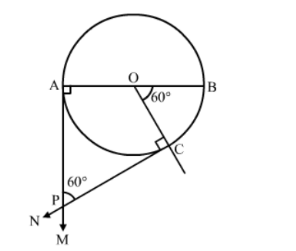# Draw a circle of radius 3.5 cm. Draw a pair of tangents to this circle,`
Question:

Draw a circle of radius 3.5 cm. Draw a pair of tangents to this circle, which are inclined to each other at an angle of 60°. Write the steps of construction.

Solution:

Steps of Construction:

Step 1. Draw a circle with centre O and radius = 3.5 cm.
Step 2. Draw any diameter AOB of this circle.

Step 3. Construct $\angle B O C=60^{\circ}$, such that the radius $O C$ meets the circle at $C$.

Step 4. Draw $\mathrm{MA} \perp A B$ and $N C \perp O C$.

Let AM and CN intersect at P.Then, PA and PC are the required tangents to the given circle that are inclined at an angle of 60o.